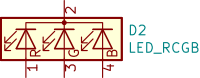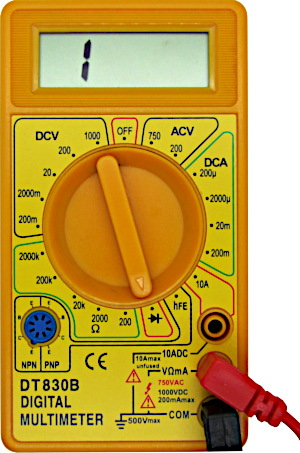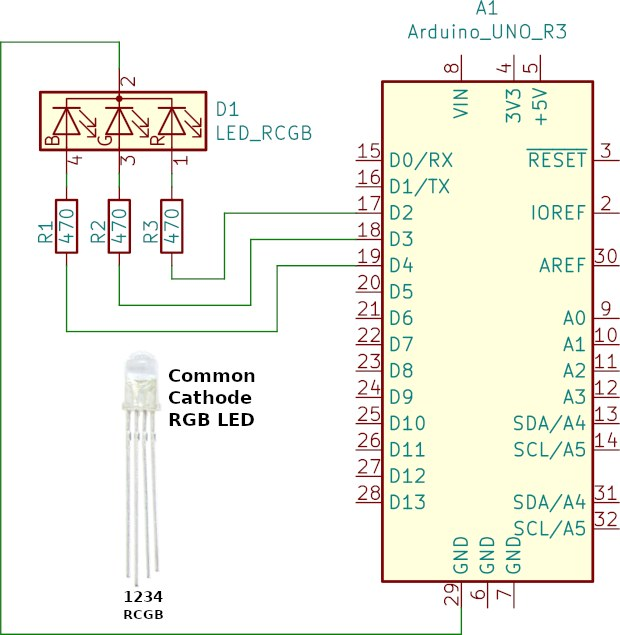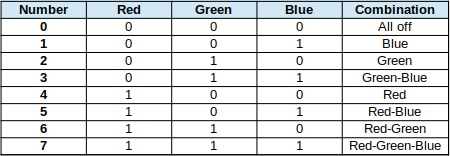Starting Electronics needs your help! Please make a donation to help cover our hosting and other costs. Click the donate button to send a donation of any amount.# Arduino RGB LED Tutorial

Created on: 1 February 2022

Arduino RGB LED tutorial for beginners in electronics. How to connect a RGB LED to an Arduino Uno or Arduino MEGA 2560 and control with code. An RGB LED is a red, green and blue light emitting diode. That is, it is three different color LEDs in a single package. Basically the idea is that any color is produced by mixing the red, green and blue colors. Use Arduino sketch code to mix the different LED colors.

Part 13 of the Arduino Tutorial for Beginners

## What is an RGB LED?

An RGB LED is a four pin LED. It is basically three LEDs in a single package with either all the LED cathodes joined together (a common cathode RGB LED). Alternatively it has all of the anodes joined together (a common anode RGB LED). The following image shows a common cathode RGB LED, although a common anode RGB LED has a similar appearance. The longest pin of the RGB LED shown below is the common cathode pin. Other pins are the red, green and blue LED anodes.

The following subsection looks at the internal wiring of RGB LEDs. After that, the next subsection looks at how to test if an RGB LED has a common anode or common cathode.

### Internal Wiring of RGB LEDs

As already mentioned, an RGB LED has either a common cathode, or a common anode. The following images show how the individual LEDs in a RGB LED are configured. For more information on normal LEDs, see the article on the basics of LEDs for beginners. That article shows what an LED anode and cathode is.

#### Common Anode RGB LED Wiring

The following image shows the internal wiring of a common anode RGB LED. That is, it is the schematic symbol or circuit diagram symbol. As can be seen in the symbol, pin number 2 is the common anode. In other words, this is where all the anodes of the LEDs join. It is important to note that not all common anode RGB LEDs have the same pin numbers. How to test the RGB LED follows the next subsection.#### Common Cathode RGB LED Wiring

The following image shows the internal wiring of a common cathode RGB LED. That is, it is the schematic symbol or circuit diagram symbol of the common cathode RGB LED. As with the common anode above, this symbol has pin 2 as the common pin. In other words, pin 2 is the common cathode, or where the three LED cathodes join. Once again, not all common cathode LEDs have the same pin numbering.### How to Test if a RGB LED is Common Anode or Common Cathode

Three methods to determine if a RGB LED is common anode or common cathode are firstly to check the datasheet, secondly test using a multimeter, and thirdly, test using a breadboard circuit.

#### RGB LED Datasheet

The primary source of information on an RGB LED, or any electronic component, is from the manufacturer’s datasheet. Every electronic manufacturer supplies datasheets for its components that contain technical details for each component. Datasheets contain the pin numbers of parts, and their electrical characteristics. For example, the forward voltages of each LED in the RGB LED and maximum current rating. The datasheet can only be found if the part number of the RGB LED is known. When a RGB LED comes in a kit or is bought without knowing the part number, test the LED to find out its pin numbering. For example, use a multimeter or breadboard circuit as described next.

#### RGB LED Multimeter Test

Most multimeters have a diode test setting. That is, a diode symbol represents the diode test. Use the diode test to determine if the RGB LED is common anode or common cathode. See the below image for a multimeter set on the diode test setting. The next paragraph describes the RGB LED multimeter test procedure.Multimeter on the Diode Test Setting
##### Common Cathode RGB LED Test

Firstly, plug the RGB LED into a breadboard to make it easier to test. Secondly, put the multimeter on to the diode test setting. Thirdly, place the probe of the black or COM multimeter lead on the long RGB LED pin. Finally place the probe of the red multimeter lead on one of the other LED pins. The LED lights up if it is a common cathode LED. Touch the red lead probe on each other LED pin in turn. Each other LED lights up assuming it is a common cathode LED. In addition to determining if the RGB LED is common cathode, the test also shows which pin is for which color LED.

##### Common Anode RGB LED Test

If the above test fails, then the RGB LED is probably a common anode device. Again, do the test with the LED plugged into a breadboard and the multimeter on the diode test setting. Place the probe of the red multimeter lead on the long RGB LED pin. Afterwards, place the probe of the black COM lead on one of the other LED pins. The LED lights up if it is a common anode device. Place the black COM lead probe on each of the other open LED pins in turn. In this way each LED color lights up in turn.

#### RGB LED Breadboard Circuit Test

Instead of using a multimeter, an RGB LED can be tested in a breadboard circuit. The following image shows the test for a common cathode RGB LED.

In the above circuit, pin 2 of the RGB LED is the long pin. Because of this, it is connected to the top GND rail of the breadboard. Connect one of the 470 ohm resistor leads to the bottom positive voltage rail. Afterwards, power the top and bottom rails from the Arduino GND and 5V pins. Connect the other end of the resistor to one of the open LED pins. That is done using the blue wire as the above image shows. If the LED lights up, the we know that it is a common cathode LED. Afterwards, test the other two open pins by connecting the blue wire to each in turn. As with the multimeter test, this reveals which color LED the pin belongs to.

If the above test fails, then power the long pin of the LED from the Arduino 5V. In addition, connect the resistor to Arduino GND. Do the same test described above. If the LED lights up, then it is a common anode LED. Test the other free pins to determine the LED colors.

## Arduino RGB LED Tutorial Circuit

The following subsections show how to connect an RGB LED to an Arduino. That is, a circuit diagram is followed by breadboard layouts for the Arduino Uno and then MEGA 2560.

### Arduino RGB LED Tutorial Circuit Diagram

The image below shows a common cathode RGB LED connected to an Arduino Uno. Be sure to change the circuit if using a RGB LED with different pin numbering. Connect the common pin to 5V instead if using a common anode RGB LED.

Use the same circuit for an Arduino MEGA 2560. That is, connect the common cathode of D1 to GND. After that, connect pin 1, 3 and 4 of D1 through 470 ohm resistors to Arduino digital pins 2, 3 and 4. Make any adjustments to the circuit if using an LED with different pin numbering.Arduino Common Cathode RGB LED Circuit Diagram

### Arduino RGB LED Tutorial Breadboard Circuits

Breadboard circuits with the above circuit are shown for the Arduino Uno and then Arduino MEGA 2560 under the subheadings that follow. The image below shows a photo of the above circuit built on breadboard and connected to an Arduino MEGA 2560 compatible board.

#### Arduino Uno RGB LED Breadboard Circuit

Build the above circuit on breadboard as shown below, if using an Arduino Uno. Whereas red wires usually represent positive voltage, in the image below, the red wire connects to the red LED anode. Likewise, the green wire connects to the green LED anode and blue to the blue LED anode. Because the RGB LED has a common cathode on pin2, pin 2 connects to Arduino GND. Connect the common pin to 5V if using a common anode LED.

#### Arduino MEGA 2560 RGB LED Breadboard Circuit

Build the above circuit on breadboard as shown below, if using an Arduino MEGA 2560. Whereas red wires usually represent positive voltage, in the image below, red represents the red LED anode. Likewise, the green wire represents the green LED anode and blue the blue LED anode. Because the RGB LED has a common cathode on pin2, pin 2 connects to Arduino GND. Connect the common pin to 5V if using a common anode LED.

## Arduino RGB LED Tutorial Sketch Code

In the following subsections are two sketches. The first simply switches the RGB LED between red, green, blue and all colors off. The second sketch switches between all possible LED on and off combinations.

### Sketch Code for Simple Switching between Red, Green and Blue

The following sketch sequences the RGB LED between red, green, blue and all off. It is nothing more than highly modified Arduino blink sketch example code, using the same functions from that sketch. This sketch is very simple, using only digital outputs to switch the red, green and blue LEDs on or off. Copy the sketch to a new sketch page in the Arduino IDE.

``````// Arduino sketch that controls a common cathode or common anode RGB LED
// Uses simple digital on/off control (not PWM)
// Displays the color sequence red, green and blue

// Swap these definitions if using a common anode RGB LED
#define LED_ON    HIGH
#define LED_OFF   LOW

// Arduino digital pins that control red, green and blue colors:
const int LED_R = 2;
const int LED_G = 3;
const int LED_B = 4;

void setup() {
// Configure pins connected to LED R, G and B as outputs
pinMode(LED_R, OUTPUT);
pinMode(LED_G, OUTPUT);
pinMode(LED_B, OUTPUT);
}

void loop() {
// Red, green and blue color sequence:
// Red on
digitalWrite(LED_R, LED_ON);
delay(1000);
// Green on
digitalWrite(LED_R, LED_OFF);
digitalWrite(LED_G, LED_ON);
delay(1000);
// Blue on
digitalWrite(LED_G, LED_OFF);
digitalWrite(LED_B, LED_ON);
delay(1000);
// All LEDs off
digitalWrite(LED_B, LED_OFF);
delay(1000);
}``````

The above sketch is set up for a common cathode RGB LED. If using a common anode RGB LED, then modify the following lines of code.

``````// Swap these definitions if using a common anode RGB LED
#define LED_ON    HIGH
#define LED_OFF   LOW``````

Change these lines as follows only if using a common anode RGB LED. As can be seen in the code, LOW and HIGH are swapped.

``````// Swap these definitions if using a common anode RGB LED
#define LED_ON    LOW
#define LED_OFF   HIGH``````

After modifying the sketch if necessary, upload it to the target Arduino board. The sketch simply switches on the red LED, then green, followed by blue. Finally, the sketch switches all LED colors off. The sequence then starts again. There is a one second delay between switching.

### Sketch Code that Switches all Color Combinations

The following sketch code switches between all possible color combinations of the RGB LED. Again, simple digital switching is used.

``````// Arduino sketch that controls a common cathode or common anode RGB LED
// Uses simple digital on/off control (not PWM)
// Displays all possible digital combinations of LED red, green and blue

// Swap these definitions if using a common anode RGB LED
#define LED_ON    HIGH
#define LED_OFF   LOW

// Arduino digital pins that control red, green and blue colors:
const int LED_R = 2;
const int LED_G = 3;
const int LED_B = 4;

void setup() {
// Configure pins connected to LED R, G and B as outputs
pinMode(LED_R, OUTPUT);
pinMode(LED_G, OUTPUT);
pinMode(LED_B, OUTPUT);
}

void loop() {
// All possible combinations of red, green and blue:
for (int i = 0; i < 8; i++) {
digitalWrite(LED_R, i & 0x04 ? LED_ON : LED_OFF);
digitalWrite(LED_G, i & 0x02 ? LED_ON : LED_OFF);
digitalWrite(LED_B, i & 0x01 ? LED_ON : LED_OFF);
delay(1000);
}
}``````

The following image shows what all possible LED color combinations are. In other words all possible digital sequences of on and off red, green and blue LEDs. Under the Red, Green and Blue headings in the table, 0 means an off LED and 1 means an on LED. The Combinations column shows the result. For example, sequence number 5 has red on, green off and blue on. This results in a red-blue color.Copy the above sketch to a new sketch page in the Arduino IDE. As with the previous sketch, modify the sketch if using a common anode LED. Upload the sketch to the target Arduino board and watch the RGB led sequence through the colors shown in the above table.